• +91 9971497814
• info@interviewmaterial.com

# Coordinate Geometry Ex-7.2 Interview Questions Answers

### Related Subjects

Question 1 : Find the coordinates of the point whichdivides the join of (- 1, 7) and (4, – 3) in the ratio 2:3.

Answer 1 :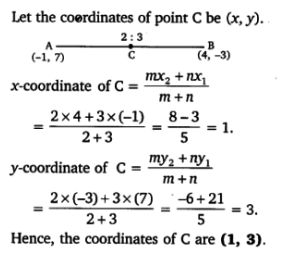Question 2 : Find the coordinates of the points oftrisection of the line segment joining (4, -1) and (-2, -3).

Answer 2 :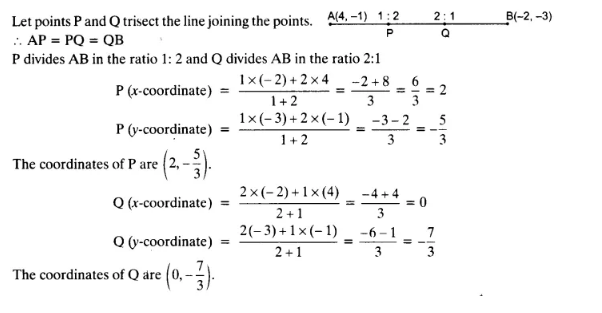Question 3 :

To conduct SportsDay activities, in your rectangular shaped school ground ABCD, lines have beendrawn with chalk powder at a distance of 1 m each.

Answer 3 : 100 flower pots have been placed at a distance of 1 m from each other along AD, as shown in the following figure. Niharika runs 1/4 th the distance AD on the 2nd line and posts a green flag. Preet runs 1/5th the distance AD on the eighth line and posts a red flag. What is the distance between both the flags? If Rashmi has to post a blue flag exactly halfway between the line segment joining the two flags, where should she post her flag?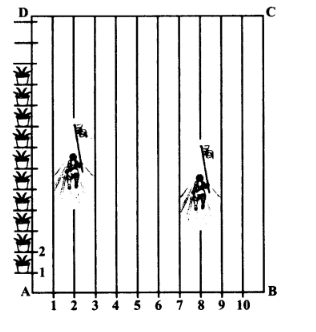Solution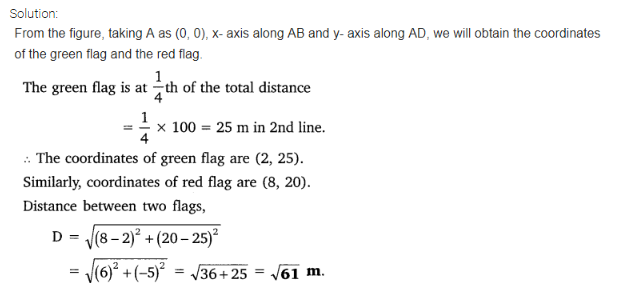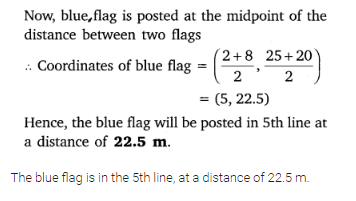Question 4 :

Find the ratio inwhich the line segment joining the points (-3, 10) and (6, – 8) is divided by(-1, 6).

Answer 4 :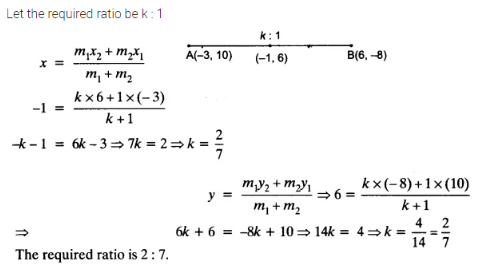Question 5 :

Find the ratio inwhich the line segment joining A (1, – 5) and B (- 4, 5) is divided by thex-axis. Also find the coordinates of the point of division.

Answer 5 :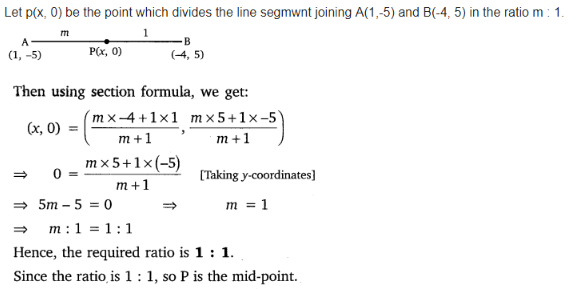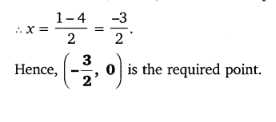Question 6 :

If (1, 2), (4, y),(x, 6) and (3, 5) are the vertices of a parallelogram taken in order,find and y.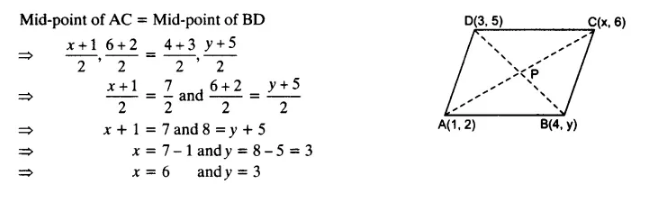Question 7 :

Find the coordinatesof a point A, where AB is the diameter of circle whose centre is (2, – 3) and Bis (1,4).

Answer 7 :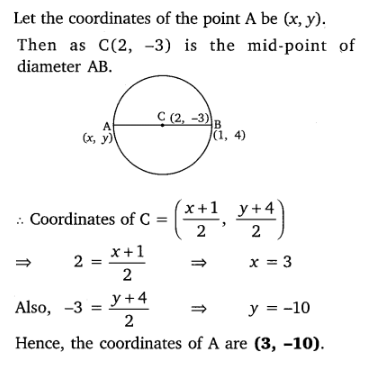Question 8 :

If A and B are (-2,-2) and (2, -4), respectively, find the coordinates of P such that AP = 3/7 AB andP lies on the line segment AB.

Answer 8 :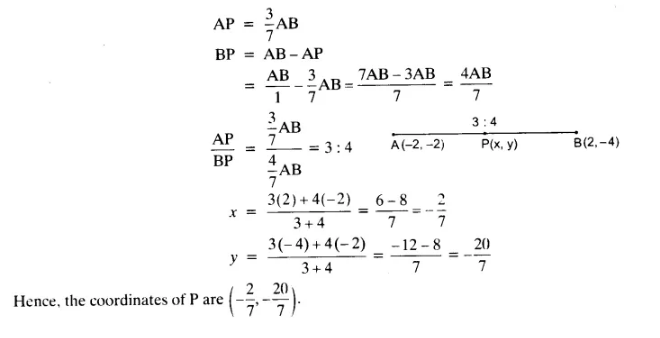Question 9 :

Find the coordinatesof the points which divide the line segment joining A (- 2, 2) and B (2, 8)into four equal parts.